Next: Laplace and Helmholtz Equation Up: HEAT EQUATION (TRANSIENT CONDUCTION) Previous: Heat Equation, 1-D Rectangular

## Heat Equation, General Case

Consider the following general boundary-value problem with vector coordinate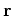: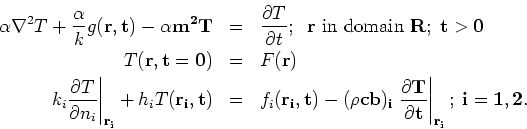The general boundary condition represents five different boundary conditions (type 1 through 5) by suitable choice of boundary parameters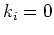or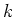;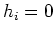or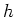;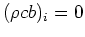or nonzero.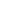Here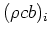represents properties of a high conductivity surface film (density, specific heat, thickness) which is thin enough that there is a negligible temperature gradient across the film and negligible heat flux parallel to the surface inside the film.

The Green's Function Solution Equation for temperature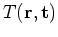is given by: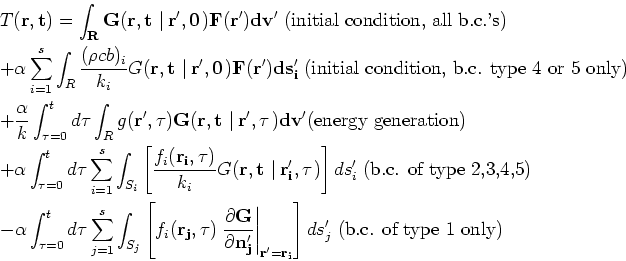This equation applies to any orthogonal coordinate system if the correct form for differential area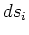and differential volume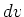are used. See the table below forandfor several body shapes in the rectangular, cylindrical, and spherical coordinate systems. Spatial derivative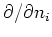denotes differentiation along the outward normal on surface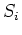,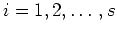where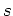represents the number of boundary conditions. The number of boundary conditionsonly includes conditions at real'' boundaries; the number of real'' boundaries does not include the boundary atfor a semi-infinite body, for example.

Table 1. Differential area and volume for the GF Solution Equation.

 Body shape coordinates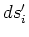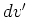plate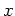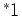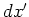rectangle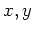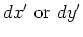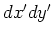parallelpiped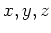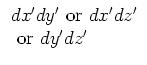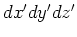infinite cylinder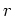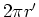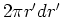thin shell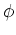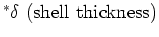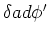finite cylinder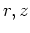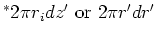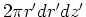wedge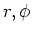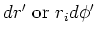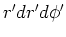sphere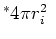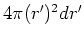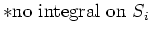Next: Laplace and Helmholtz Equation Up: HEAT EQUATION (TRANSIENT CONDUCTION) Previous: Heat Equation, 1-D Rectangular
2004-01-31